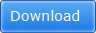## Pages

### CBSE MATH STUDY: Linear Equation solved hots questions

Q. Places A and B are 100kms apart on a highway. One car starts from A and another starts from B at the same time. If the car travels in the same direction at different the speeds, they meet in 5hrs.If they travelled towards each other they meet in 1hr.What are the speeds of the two cars?

Let the speed of 1st car and 2nd car be x km/h and y km/h.
Respective speed of both cars while they are travelling in same direction = (x-y) km/h
Respective speed of both cars while they are travelling in opposite directions i.e., travelling towards each other = ((x + y) km/h
According to the given information,
First case, in the same direction A and B meet in 5hrs
Distance cover to meet = distance x time
5 (x-y) = 100 Þ x – y =100/5 Þ x- y = 20 Þ x = 20+y
Second case, If they travelled towards each other they meet in 1hr,
1(x+y) = 100 Þ 20+y +y =100
2y= 100-20=80
Y=80/2=40
So, x = 100 – 40= 60
Hence, speed of one car = 60 km/h and speed of other car = 40 km/h

Q. If two the lines representing equations are overlapped on graph then what is the intersection point?
If two lines overlap each other, then there are infinite points of intersections. Hence, two coincident lines have infinite points of intersection.

Q. Divide 123 rupees A and B so that B will get as many 25 paise coins as 50 paise coins a gets.
Let the number of coins with both be x
Then money with A = x × 50 paise = Rs 0.50 x
and money with B = x × 25 paise = Rs 0.25 x
Now A and B have Rs 123 in total 0.50 x + 0.25 x = 123 0.75 x = 123 X = 123/0.75=164
Hence money with A = Rs 0.50 × 164 = Rs 82 And money with B = Rs 0.25 × 164 = Rs 41

Q. A bag contains a number of 10 paise coins, 3 times as many 25 paise coins as 10 paise coins and five more 50 paise coins, than 25 paise coins. If the total value is Rs 120. How many 10 paise coins are there?